# Math Functions in oracle | Math Functions | Numeric OR Mathematical Function - oracle tutorial - sql tutorial

## What is Math Functions in oracle ?

• Oracle SQL Mathematical functions are used primarily for numeric manipulation and/or mathematical calculations.
• The following table defines the numeric functions.

## ABS()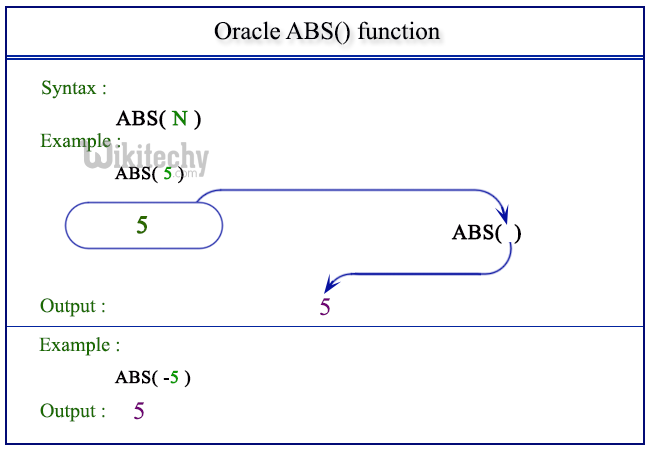## ACOS()

• Returns the arccosine of numeric expression. Returns NULL if the value is not in the range -1 to 1.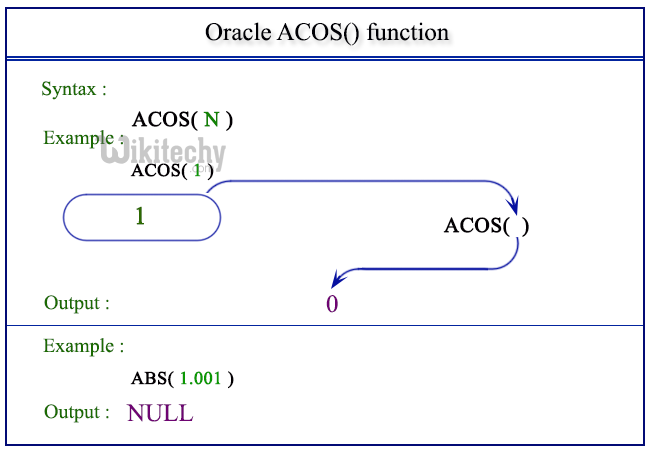## ASIN()

• Returns the arcsine of numeric expression. Returns NULL if value is not in the range -1 to 1.## ATAN()

• Returns the arctangent of numeric expression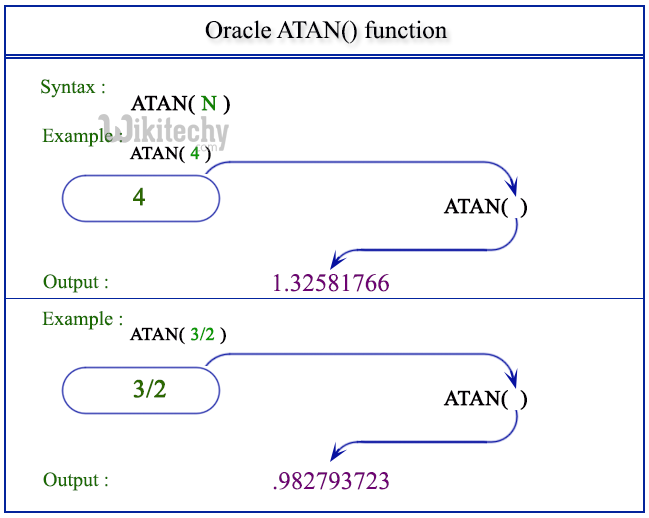## ATAN2()

• Returns the arctangent of the two variables passed to it

## BIT_AND()

• Returns the bitwise AND all the bits in expression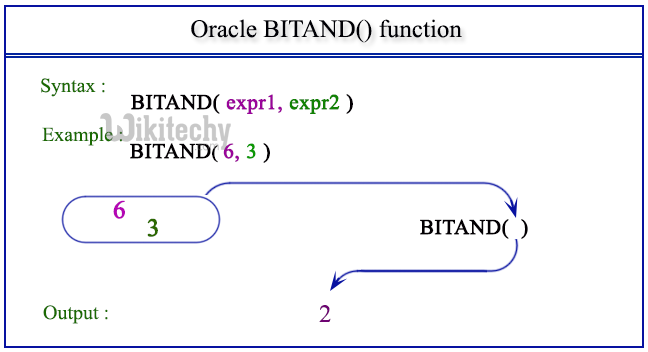## BIT_COUNT()

• Returns the string representation of the binary value passed to it

## BIT_OR()

• Returns the bitwise OR of all the bits in the passed expression

## CEIL()

• Returns the smallest integer value that is not less than passed numeric expression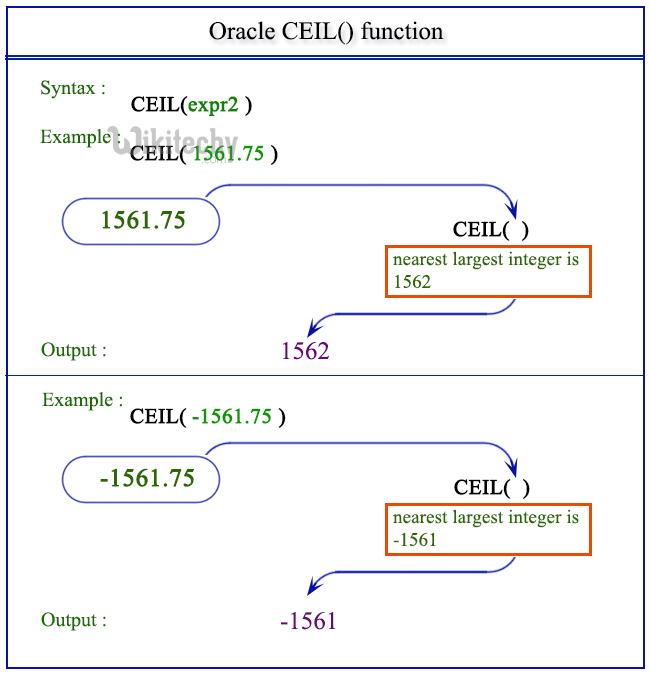## CEILING()

• Returns the smallest integer value that is not less than passed numeric expression

## CONV()

• Convert numeric expression from one base to another

## COS()

• Returns the cosine of passed numeric expression. The numeric expression should be expressed in radians

## COT()

• Returns the cotangent of passed numeric expression.

## DEGREES()

• Returns numeric expression converted from radians to degrees.

## EXP()

• Returns the base of the natural logarithm (e) raised to the power of passed numeric expression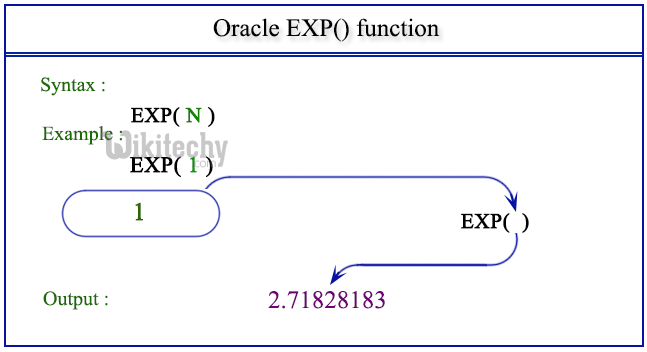## FLOOR()

• Returns the largest integer value that is not greater than passed numeric expression

## FORMAT()

• Returns a numeric expression rounded to a number of decimal places

## GREATEST()

• Returns the largest value of the input expressions.

## LEAST()

• Returns the minimum-valued input when given two or more

## LOG()

• Returns the natural logarithm of the passed numeric expression## LOG10()

• Returns the base-10 logarithm of the passed numeric expression

## MOD()

• Returns the remainder of one expression by diving by another expression.## PI()

• Returns the value of pi.

## POW()

• Returns the value of one expression raised to the power of another expression

## POWER()

• Returns the value of one expression raised to the power of another expression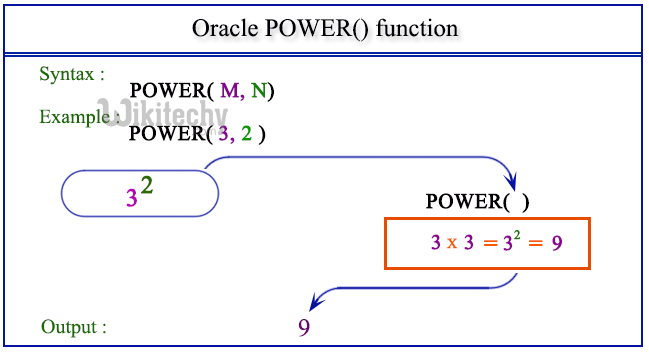• Returns the value of passed expression converted from degrees to radians

## ROUND()

• Returns numeric expression rounded to an integer. Can be used to round an expression to a number of decimal points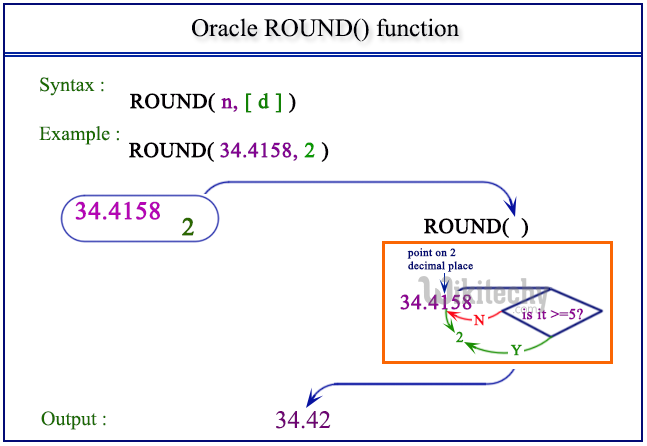## SIN()

• Returns the sine of numeric expression given in radians.

## SQRT()

• Returns the non-negative square root of numeric expression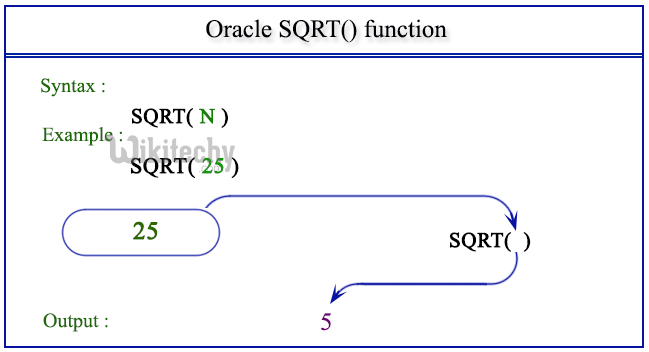## STD()

• Returns the standard deviation of the numeric expression

## TAN()

• Returns the tangent of numeric expression expressed in radians.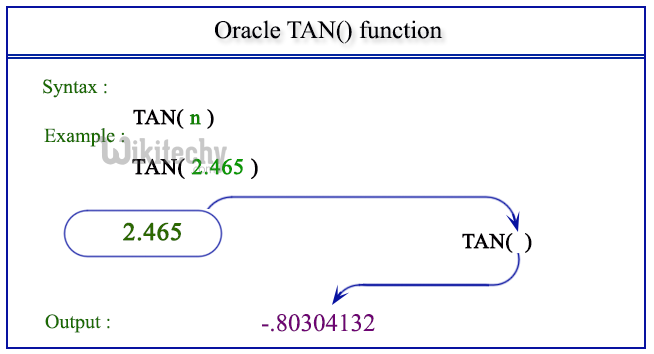## TRUNCATE()

• Returns numeric exp1 truncated to exp2 decimal places. If exp2 is 0, then the result will have no decimal point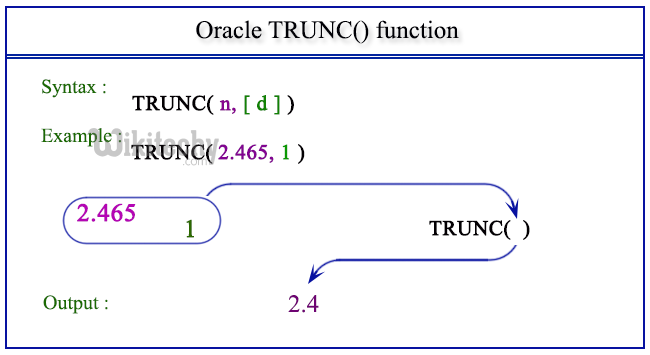## Max Function:

• The Oracle/PLSQL MAX function returns the maximum value from the particular or multiple columns.

## Sample Database

• For better understanding we are using wikitechy_employee table.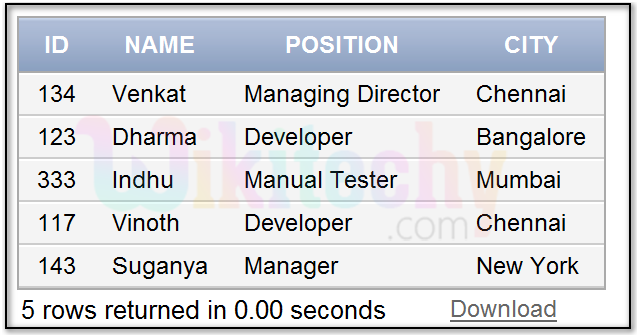## Code Explanation: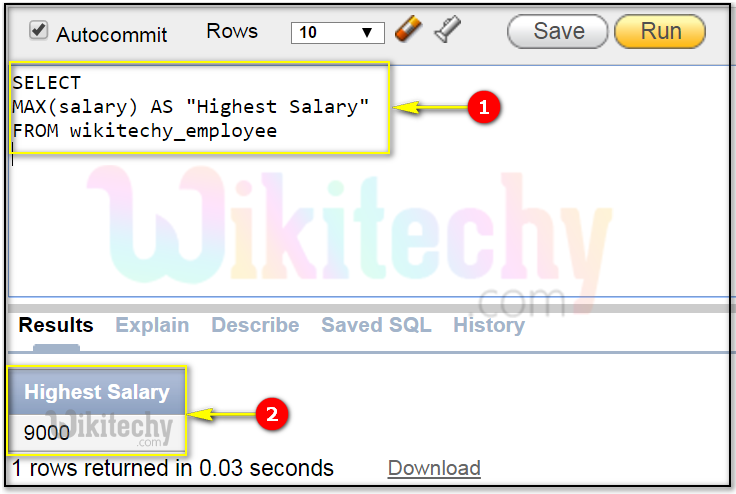1. We have aliased the MAX(salary) expression as "Highest Salary". As a result, "Highest Salary" will display as the field name when the result set is returned.
2. You can find the maximum salary as highest salary.